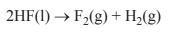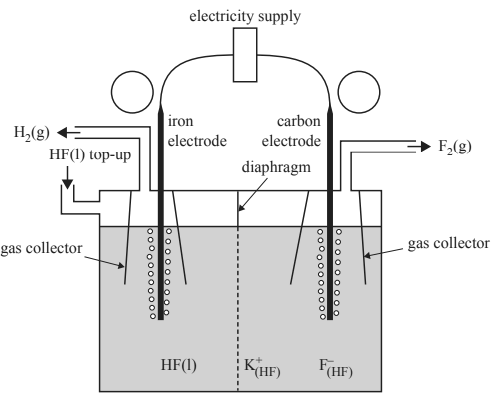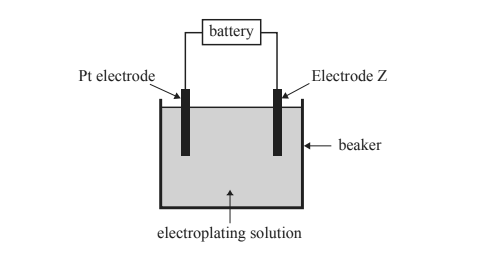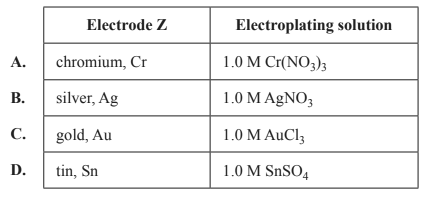Electrolysis (2017 VCE) 1) Fluorine, F2, gas is the most reactive of all non-metals. Anhydrous liquid hydrogen fluoride, HF, can be electrolysed to produce F2 and hydrogen, H2, gases. Potassium fluoride, KF, is added to the liquid HF to increase electrical conductivity. The equation for the reaction isF2 is used to make a range of chemicals, including sulfur hexafluoride, SF6, an excellent electrical insulator, and xenon difluoride, XeF2, a strong fluorinating agent. The diagram below shows an electrolytic cell used to prepare F2 gas.Liquid HF, like water, is an excellent solvent for ionic compounds. In the same way that water molecules in an aqueous solution form the ions K+(aq) and F-(aq), when KF is dissolved in HF, the K+ and F- ions form ions that are written as K+ (HF) and F- (HF). a. Label the polarities of each electrode in the circles provided on the diagram above. Solutionb. Write the equation for the half-reaction occurring at the anode. Solutionc. Suggest why the diaphragm, shown in the diagram above, is important for the safe operation of the electrolytic cell. Solutiond. Explain why the carbon electrode cannot be replaced with an iron electrode. Solutione. Calculate the volume of F2 gas, measured at standard laboratory conditions (SLC), that would be produced when a current of 1.50 A is passed through the cell for 2.00 hours. Solution2) The diagram below shows the basic set-up of an electroplating cell.Initially the cell is set up with a lead, Pb, electrode as Electrode Z and 1.0 M lead nitrate, Pb(NO3)2, as the electroplating solution. The cell runs for a set time and current, with 1.0 g of Pb deposited onto Electrode Z. Four subsequent electroplating cells are set up, each containing a platinum, Pt, electrode, a different Electrode Z and an appropriate 1.0 M electroplating solution. These four electroplating cells are operated for the same time and at the same current as the original Pb electroplating cell. Which combination of Electrode Z and electroplating solution would be expected to deposit more metal by mass onto Electrode Z than the original Pb electroplating cell?Solution